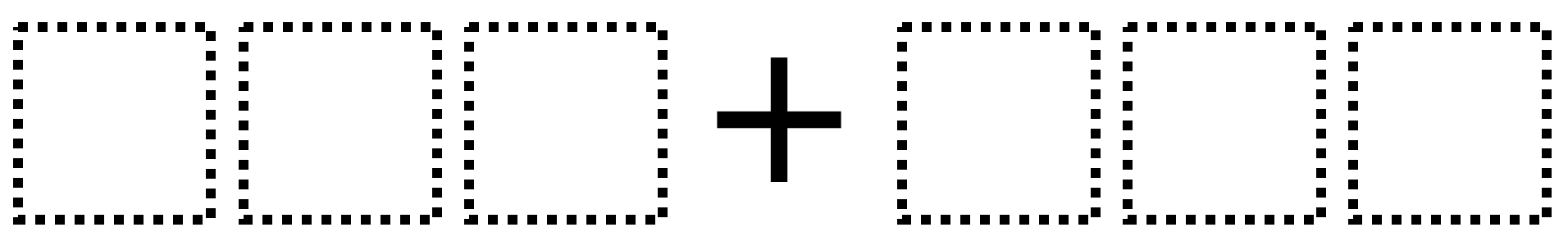# Sum to 1,000 – Two Addends

Directions: Arrange the digits 1-6 into two 3-digit whole numbers. Make the sum as close to 1000 as possible.### Hint

Which digits will be the most helpful to focus on to make the solution as close to 1,000 as possible?

993 (multiple ways to create the solution)

Source: Ian Kerr

## Time Twister

Directions: Using the digits 0 to 9, at most one time each, create three different …

1.Not able to pick this up in a PDF. Is there a trick to it?

2.Still not working as a PDF! 🙁

3.Can’t get it to work either……

4.Last week when I was online at the site, it allowed me to save to google drive as a pdf. Where did that option go? Please help!

•Hi Sondra. I just added it back. It was buggy and I thought no one used it. Glad to be wrong and sorry for the inconvenience.

5.6.400+600=1000

7.Daniel J. Lombardo

Nico 500+500=1000

8.500+500

•1.666+334
2.555+445
Two ways to create 1,000 using no zeros

•ashton nguyen, you can’t have any zeros.

9.10.444 + 556 = 1000.

•I believe you’re supposed to use *all* the digits 1 – 6 in forming the two numbers. (And, you can only use each digit one time…)

11.•I’m posting a “general” comment, b/c so many responses/comments are erroneously doing one of the following:

1) Including decimal values (problem states that the two numbers must be *whole* numbers)
2) Including the digit zero (not allowed)
3) Failing to use each of the digits 1 – 6 one time each.

12.500+500=1000

13.14.IM Jr but my name is really YAEL SANTOS

15.500+500

16.17.18.500+500=1,000

19.900+100=1000

•400+600=1,000

•captain obvious jr.

you cant have zeros, Sai.M

20.666+334=1,000

21.one hundred plus nine hundred equals one thousand
it is so easy.

22.The answer given above states that the closest sum to 1000 that you can form from the digits 1 – 6 is 993.

I believe there are 16 different ways to do so, with one example being: 431 + 562.

23.561 + 432 = 993
So close! It is not possible to get to 1,000.